Question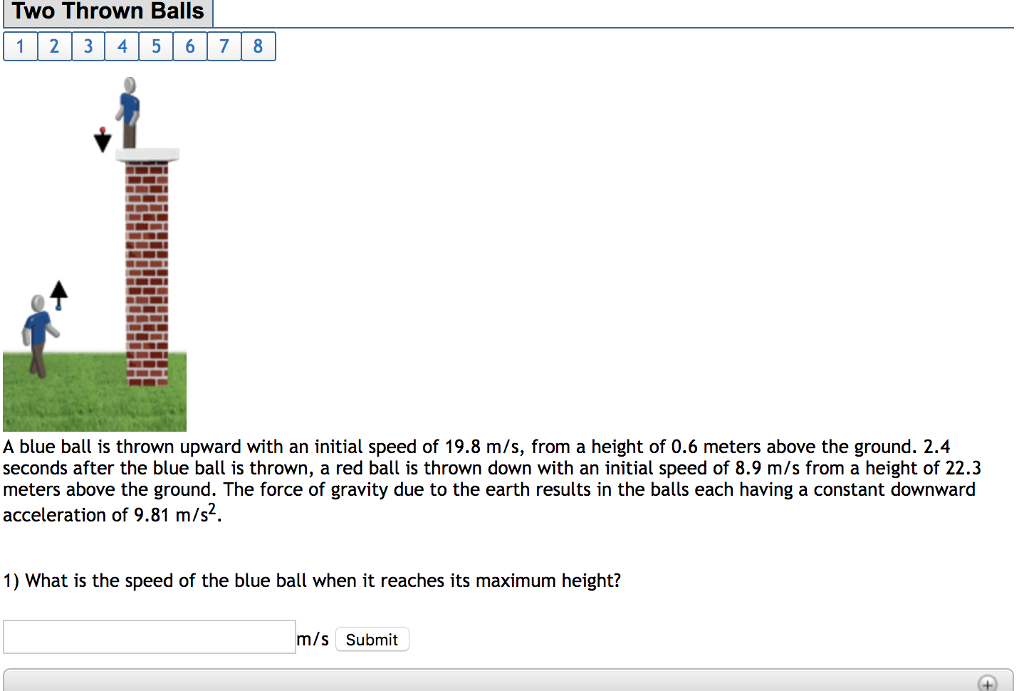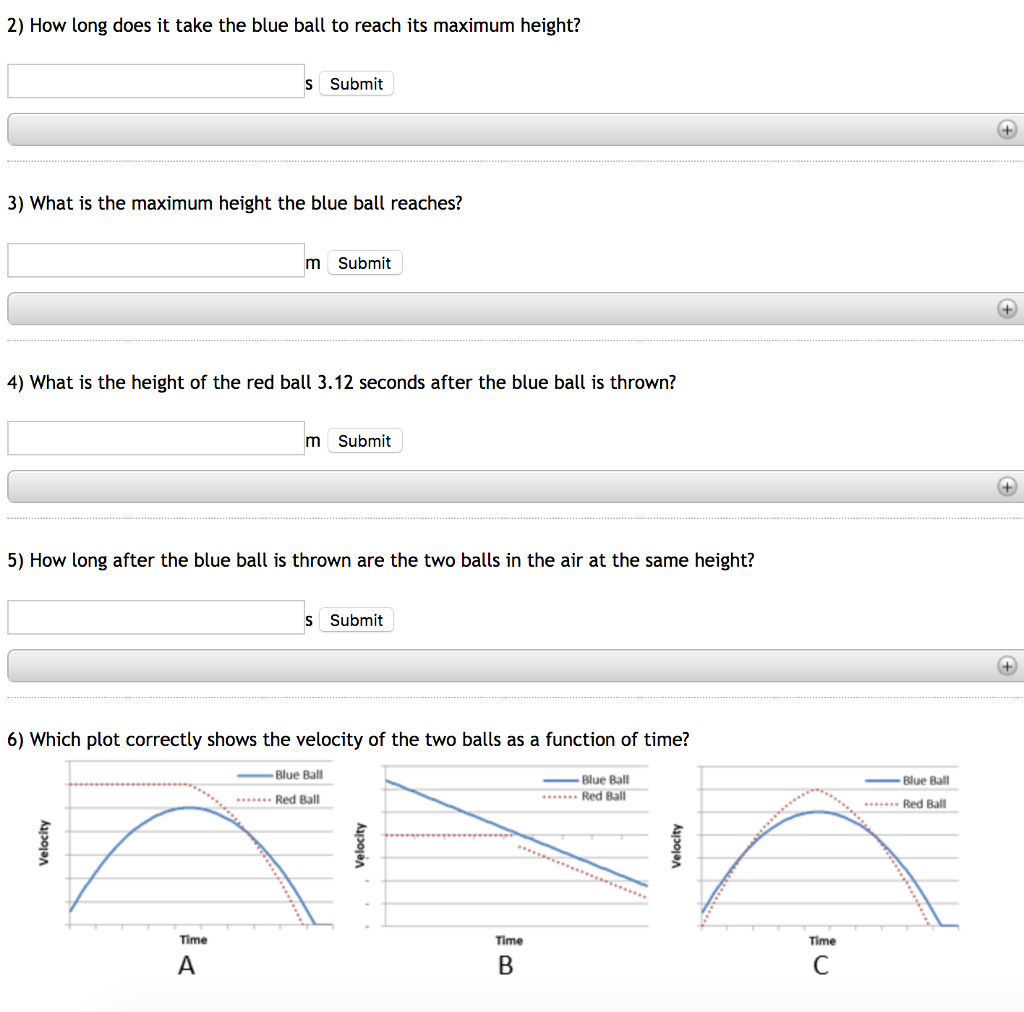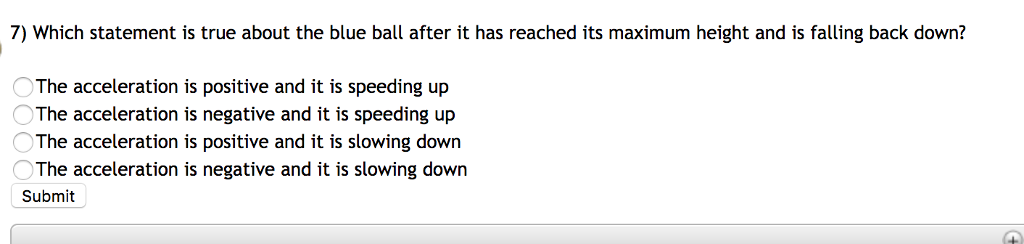Allowed to answer only 4 sub parts at a time According to the HOMEWORKLIB RULES.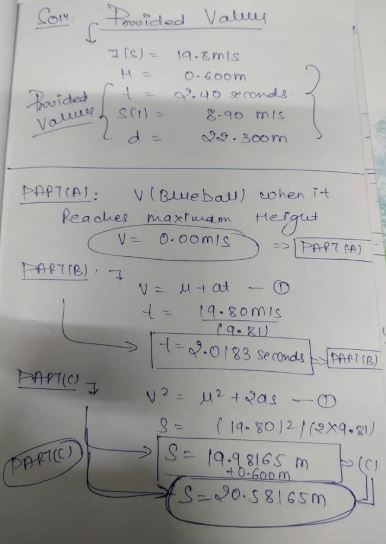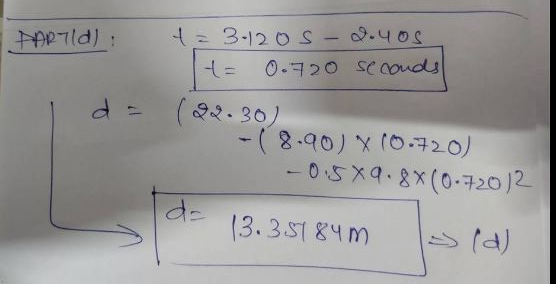#### Earn Coins

Coins can be redeemed for fabulous gifts.

Similar Homework Help Questions
• ### Two throw A blue ball is thrown upward with an initial speed of 22.2 m/s, from...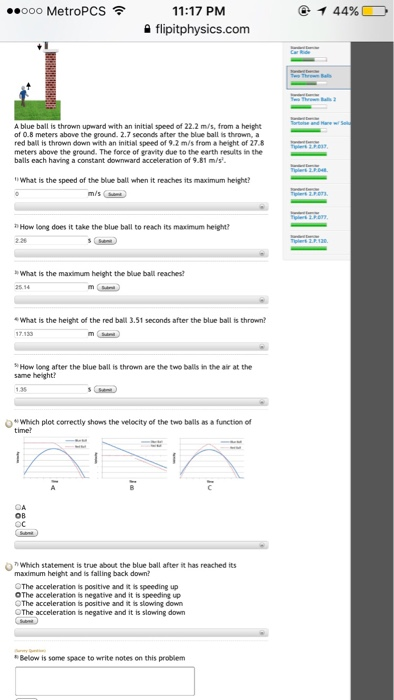Two throw A blue ball is thrown upward with an initial speed of 22.2 m/s, from a height of 0.8 meters above the ground. 2.7 seconds after the blue ball is thrown, a red ball is thrown down with an initial speed of 9.2 m/s from a height of 27.8 meters above the ground. The force of grandly due to the earth results in the balls each having a constant downward acceleration of 9.81 m/s^2. What is the speed of...

• ### Please help with the last few parts A blue ball is thrown upward with an initial...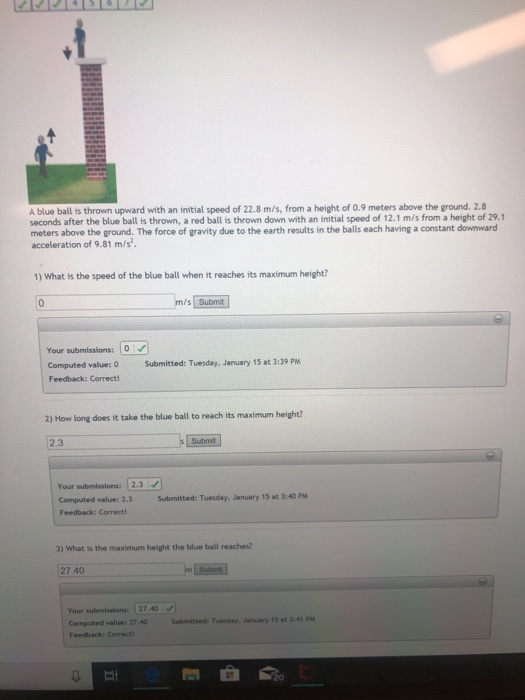Please help with the last few parts A blue ball is thrown upward with an initial speed of 22.8 m/s, from a height of 0.9 meters above the ground. 2.8 seconds after the blue ball is thrown, a red ball is thrown down with an initial speed of 12.1 m/s from a height of 29.1 meters above the ground. The force of gravity due to the earth results in the balls each having a constant downward acceleration of 9.81 m/s...

• ### A blue ball is thrown upward with an initial speed of 19.8 m/s, from a height of 0.5 meters above the ground

A blue ball is thrown upward with an initial speed of 19.8 m/s, from a height of 0.5 meters above the ground. 2.4 seconds after the blue ball is thrown, a red ball is thrown down with an initial speed of 7.9 m/s from a height of 22.5 meters above the ground. The force of gravity due to the earth results in the balls each having a constant downward acceleration of 9.81 m/s2. What is the maximum height the blue...

• ### A blue ball is thrown upward with an initial speed of 24.1 m/s, from a height...

A blue ball is thrown upward with an initial speed of 24.1 m/s, from a height of 0.8 meters above the ground. 2.9 seconds after the blue ball is thrown, a red ball is thrown down with an initial speed of 10.4 m/s from a height of 31.4 meters above the ground. The force of gravity due to the earth results in the balls each having a constant downward acceleration of 9.81 m/s2. What is the height of the red...

• ### A blue ball is thrown upward with an initial speed of 23.9 m/s, from a height...

A blue ball is thrown upward with an initial speed of 23.9 m/s, from a height of 0.5 meters above the ground. 2.9 seconds after the blue ball is thrown, a red ball is thrown down with an initial speed of 7.5 m/s from a height of 31.5 meters above the ground. The force of gravity due to the earth results in the balls each having a constant downward acceleration of 9.81 m/s^2. How long after the blue ball is...

• ### A blue ball is thrown upward with an initial speed of 20 m/s, from a height...

A blue ball is thrown upward with an initial speed of 20 m/s, from a height of 0.8 meters above the ground. 2.4 seconds after the blue ball is thrown, a red ball is thrown down with an initial speed of 5.3 m/s from a height of 22.4 meters above the ground. The force of gravity due to the earth results in the balls each having a constant downward acceleration of 9.81 m/s2. 1) How long after the blue ball...

• ### A red ball is thrown down with an initial speed of 1.3 m/s from a height...

A red ball is thrown down with an initial speed of 1.3 m/s from a height of 26 meters above the ground. Then, 0.4 seconds after the red ball is thrown, a blue ball is thrown upward with an initial speed of 23.9 m/s, from a height of 1 meters above the ground. The force of gravity due to the earth results in the balls each having a constant downward acceleration of 9.81 m/s2. What is the speed of the...

• ### A red ball is thrown down with an initial speed of 1.2 m/s from a height...

A red ball is thrown down with an initial speed of 1.2 m/s from a height of 25 meters above the ground. Then, 0.6 seconds after the red ball is thrown, a blue ball is thrown upward with an initial speed of 23.8 m/s, from a height of 0.8 meters above the ground. The force of gravity due to the earth results in the balls each having a constant downward acceleration of 9.81 m/s^2. 1)What is the speed of the...

• ### A red ball is thrown down with an initial speed of 1.2 m/s from a height...

A red ball is thrown down with an initial speed of 1.2 m/s from a height of 26 meters above the ground. Then, 0.4 seconds after the red ball is thrown, a blue ball is thrown upward with an initial speed of 23.8 m/s, from a height of 1 meters above the ground. The force of gravity due to the earth results in the balls each having a constant downward acceleration of 9.81 m/s2. 1) What is the speed of...

• ### Two identical balls are thrown vertically upward. The second ball is thrown with an initial speed...

Two identical balls are thrown vertically upward. The second ball is thrown with an initial speed that is twice that of the first ball. How does the maximum height of the two balls compare? The maximum heights of the two balls are equal. The maximum height of the second ball is two times that of the first ball. The maximum height of the second ball is four times that of the first ball. The maximum height of the second ball...